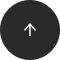MySQL

# How to Group by Year in SQL

#### Operators:

GROUP BY, EXTRACT, OVER, PARTITION BY, SUM

#### Problem:

You want to group your data by year.

#### Example I:

One of the columns in your data is `transaction_date`. It contains a date. You would like to group all your data by year and calculate the total money earned each year.

The `data` table looks like this:

transaction_datemoney
2018-03-251700
2019-09-12100
2018-07-141200
2018-01-05400
2019-06-082000
2020-03-061500

#### Solution 1 (displaying the year and the money earned):

```SELECT
EXTRACT(year FROM transaction_date) AS year,
SUM(money) AS money_earned
FROM data
GROUP BY EXTRACT(year FROM transaction_date);
```

The result is:

yearmoney_earned
20201500
20192100
20183300

#### Solution 2 (displaying the complete date, the year, and the money earned in the corresponding year):

```SELECT
transaction_date AS transaction_date,
EXTRACT(year FROM transaction_date) AS year,
SUM(money) OVER(PARTITION BY EXTRACT(year FROM transaction_date)) AS money_earned
FROM data;
```

The result is:

transaction_dateyearmoney_earned
2018-03-2520183300
2018-07-1420183300
2018-01-0520183300
2019-09-1220192100
2019-06-0820192100
2020-03-0620201500

#### Discussion:

In this example it's assumed that you don't have the `year` column. Instead you have the column with complete dates.

First, you need to retrieve a year from the date. You can use the `EXTRACT(part FROM date)` function to do it. In your case, you'd like to extract the year, so the `part` is `year`. The `date` is the column which contains the dates – the `transaction_date` column. It's a good idea to rename the column to year afterwards. If you want to learn more about the `EXTRACT` function and how to retrieve different parts from the date, you can find it here.

If you'd like to display just the year and the total money earned in this year, you can use a `GROUP BY`. The first selected column is the year extracted from the date. The second column is the aggregate function `SUM(money)`. At the end of the query you need a `GROUP BY EXTRACT(year FROM transaction_date)` or, simpler, `GROUP BY 1 (since EXTRACT(year FROM transaction_date)` is the first column.)

If you'd like to display more columns, you need a window function (Solution 2). After `SUM(money)` you write the `OVER()` clause and, since you'd like to calculate the sum for each year, use `PARTITION BY EXTRACT(year FROM transaction_date)` inside it. Note that you don't yet have the year column when calculating the sum, so `PARTITION BY year` won't work – you would get an error `'column "year" does not exist'`. You can read more about the window functions in this article.

#### Example II:

One of the columns in your data is `year`. You would like to group all your data by this column and calculate the total money earned each year.

The `data` table looks like this:

yearmonthdaymoney
20183251700
2019912100
20187141200
201815400
2019682000
2020361500

#### Solution 1 (displaying the year and the money earned):

```SELECT
year,
SUM(money) AS money_earned
FROM data
GROUP BY year;
```

The result is:

yearmoney_earned
20201500
20183300
20192100

#### Solution 2 (displaying year, month, day and the money earned in the corresponding year):

```SELECT
year,
month,
day,
SUM(money) OVER(PARTITION BY year) AS money_earned
FROM data;
```

The result is:

yearmonthdaymoney_earned
20183253300
20187143300
2018153300
20199122100
2019682100
2020361500

#### Discussion:

In this example it's assumed that you already have the `year` column.

If you'd like to display the year and the total money earned in this year, a simple `GROUP BY` is enough. If you don't feel comfortable with the concept of `GROUP BY`, take a look here where we explain it. You simply use the aggregate function (here: `SUM`) with the correct column and at the end of the query you group by `year`. You can rename the column using the `AS` keyword with a new name.

It's more complicated if you'd also like to display some other columns. Then you need the solution using a window function (Solution 2). You should use the aggregate function with the appropriate column (here: `SUM(money)`) and write the `OVER()` clause afterwards. In this clause, you should use `PARTITION BY` with the column by which you'd like to group. That's how you get:

```SUM(money) OVER(PARTITION BY year)
```

In this solution, you don't use a `GROUP BY` clause.